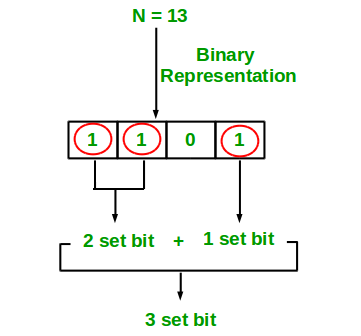# C/C++ Program to Count set bits in an integer

• Last Updated : 02 Jan, 2019

Write an efficient program to count number of 1s in binary representation of an integer.

Examples :

```Input : n = 6
Output : 2
Binary representation of 6 is 110 and has 2 set bits

Input : n = 13
Output : 3
Binary representation of 11 is 1101 and has 3 set bits
```## Recommended: Please solve it on “PRACTICE ” first, before moving on to the solution.

1. Simple Method Loop through all bits in an integer, check if a bit is set and if it is then increment the set bit count. See below program.

## C

 `#include `` ` `/* Function to get no of set bits in binary``   ``representation of positive integer n */``unsigned ``int` `countSetBits(unsigned ``int` `n)``{``    ``unsigned ``int` `count = 0;``    ``while` `(n) {``        ``count += n & 1;``        ``n >>= 1;``    ``}``    ``return` `count;``}`` ` `/* Program to test function countSetBits */``int` `main()``{``    ``int` `i = 9;``    ``printf``(``"%d"``, countSetBits(i));``    ``return` `0;``}`

Output:

```2
```

Recursive Approach :

## C++

 `// Cpp implementation of recursive``// approach to find the number``// of set bits in binary representation``// of positive integer n``#include ``using` `namespace` `std;`` ` `// recursive function to count set bits``int` `countSetBits(``int` `n)``{``    ``// base case``    ``if` `(n == 0)``        ``return` `0;`` ` `    ``else`` ` `        ``// if last bit set add 1 else add 0``        ``return` `(n & 1) + countSetBits(n >> 1);``}`` ` `// driver code``int` `main()``{``    ``// get value from user``    ``int` `n = 9;`` ` `    ``// function calling``    ``cout << countSetBits(n);`` ` `    ``return` `0;``}`` ` `// This code is contributed by Raj.`

Output:

```2
```

Please refer complete article on Count set bits in an integer for more details!

My Personal Notes arrow_drop_up